### Asymptotic Notations: Big O, Big Omega and Big Theta Explained (With Notes)

Either you can download the notes in pdf (Link is at the end of the page) or you can read them on this site itself.

#### Asymptotic Notations

Asymptotic notation gives us an idea about how good a given algorithm is compared to some other algorithm.

Let us see the mathematical definition of “order of” now,

Primarily there are three types of widely used asymptotic notations.

1. Big oh notation ( O )
2. Big omega notation ( Ω )
3. Big theta notation ( θ ) – Widely used one

Big oh notation ( O )

Big oh notation is used to describe asymptotic upper bound.

Mathematically, if f(n) describes running time of an algorithm; f(n) is O(g(n)) if there exist positive constants C and no such that,

O ≤ f(n) ≤ c g(n)           for all n ≥ no              [It is used to give upper bound on a function]

If a function is O(n), it is automatically O(n2) as well!

Graphic example for Big oh ( O ):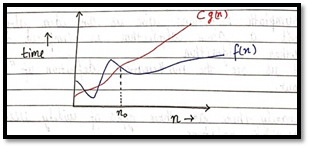Big Omega Notation ( Ω )

Just like O notation provides an asymptotic upper bound, Ω notation provides asymptotic lower bound. Let f(n) define the running time of an algorithm;

F(x) is said to be Ω (gx) if there exist positive constants C and no such that,

O ≤ c g(x) ≤ f(x)                 for all n ≥ no                 (used to give lower bound on a function)

If a function is Ω (n2) it is automatically Ω (n) as well.

Graphic example for Big Omega (Ω)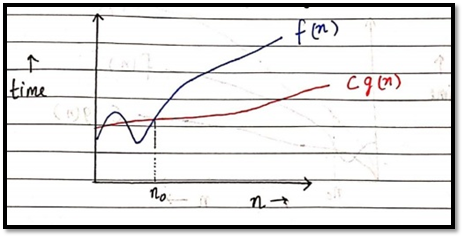Big theta notation ( θ )

Let f(x) define running time of an algorithm

F(x) is said to be θ (gx) if f(x) is O (g(x)) and f(x) is Ω (g(x)).

Mathematically,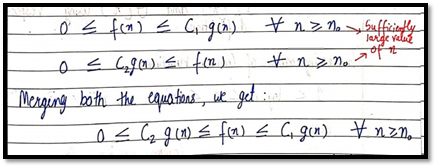The equation simply means there exist positive constants C1 and C2 such that f(x) is sandwiched between C2 g(x) and C1 g(x).

Graphic example of Big theta ( θ )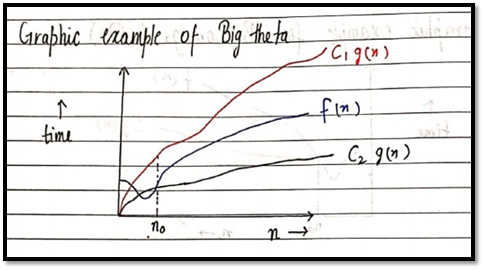Which one of these to use?

Since Big theta gives a better picture of run time for a given algorithm, most of the interviewers expect you to provide an answer in terms of Big theta when they say “order of”.

Quick Quiz: Prove that n2+n+1 is O(n3), Ω(n2), and θ(n2) using respective definitions.

Increasing order of common runtimes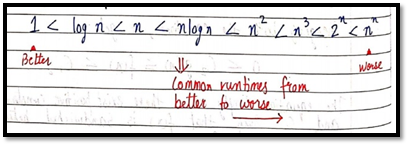prateekwh 1 month, 2 weeks ago
`bhai mujhe kuch bhi kyo smjh me nhi aa rha hai bhai mujhe ye smjhana hai please bhai Help `mohit01 1 month, 2 weeks ago
`Sir I want to ask Machine Learning ki playlist me complete course h ya abhi kuch chize bachi h aur kya wo sb in future cover kroge aap`

#### Resources

1. Asymptotic Notations -

#### Course Announcements

Any Course related announcements will be posted here